[Introduction]  [Signal arithmetic]  [Signals and noise]   [Smoothing]   [Differentiation]  [Peak Sharpening]  [Harmonic analysis]   [Fourier convolution]  [Fourier deconvolution]  [Fourier filter]  [Wavelets]   [Peak area measurement]  [Linear Least Squares]  [Multicomponent Spectroscopy]  [Iterative Curve Fitting]  [Hyperlinear quantitative absorption spectrophotometry] [Appendix and Case Studies]  [Peak Finding and Measurement]  [iPeak]   [iSignal]  [Peak Fitters]   [iFilter]  [iPower]  [List of downloadable software]  [Interactive tools]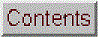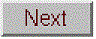## Fourier Deconvolution

Fourier deconvolution is the converse of Fourier convolution in the sense that division is the converse of multiplication. If you know that m times x equals n, where m and n are known but x is unknown, then x equals n divided by m. Conversely if you know that m convoluted with x equals n, where m and n are known but x is unknown, then x equals m deconvoluted from n.

In practice, the deconvolution of one signal from another is usually performed by point-by-point division of the two signals in the Fourier domain, that is, dividing the Fourier transforms of the two signals point-by-point and then inverse-transforming the result. Fourier transforms are usually expressed in terms of complex numbers, with real and imaginary parts representing the sine and cosine parts. If the Fourier transform of the first signal is a + ib, and the Fourier transform of the second signal is c + id, then the ratio of the two Fourier transforms is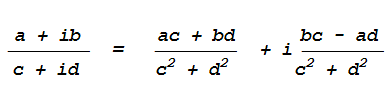by the rules for the division of complex numbers. Many computer languages will perform this operation automatically when the two quantities divided are complex.

Note: The word "deconvolution" can have two meanings, which can lead to confusion. The Oxford dictionary defines it as "A process of resolving something into its constituent elements or removing complication in order to clarify it", which in one sense applies to Fourier deconvolution. But the same word is also sometimes used for the process of resolving or decomposing a set of overlapping peaks into their separate additive components by the technique of iterative least-squares curve fitting of a proposed peak model to the data set. However, that process is actually conceptually distinct from Fourier deconvolution, because in Fourier deconvolution, the underlying peak shape is unknown but the broadening function is assumed to be known; whereas in iterative least-squares curve fitting it's just the reverse: the peak shape must be known but the width of the broadening process, which determines the width and shape of the peaks in the recorded data, is unknown. Thus the term "spectral deconvolution" is ambiguous: it might mean the Fourier deconvolution of a response function from a spectrum, or it might mean the decomposing of a spectrum into its separate additive peak components. These are different processes; don't get them confused.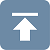The practical significance of Fourier deconvolution in signal processing is that it can be used as a computational way to reverse the result of a convolution occurring in the physical domain, for example, to reverse the signal distortion effect of an electrical filter or of the finite resolution of a spectrometer. In some cases the physical convolution can be measured experimentally by applying a single spike impulse ("delta") function to the input of the system, then that data used as a deconvolution vector. Deconvolution can also be used to determine the form of a convolution operation that has been previously applied to a signal, by deconvoluting the original and the convoluted signals. These two types of application of Fourier deconvolution are shown in the two figures below.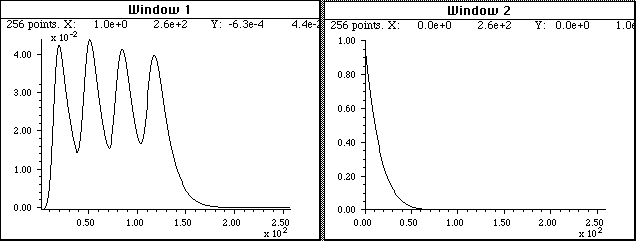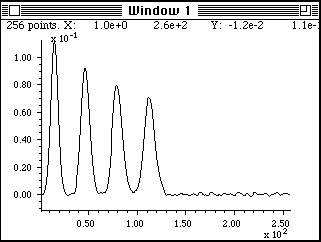Fourier deconvolution is used here to remove the distorting influence of an exponential tailing response function from a recorded signal (Window 1, top left) that is the result of an unavoidable RC low-pass filter action in the electronics. The response function (Window 2, top right) must be known and is usually either calculated on the basis of some theoretical model or is measured experimentally as the output signal produced by applying an impulse (delta) function to the input of the system. The response function, with its maximum at x=0, is deconvoluted from the original signal . The result (bottom, center) shows a closer approximation to the real shape of the peaks; however, the signal-to-noise ratio is unavoidably degraded compared to the recorded signal, because the Fourier deconvolution operation is simply recovering the original signal before the low-pass filtering, noise and all. (Matlab/Octave script)Note that this process has an effect that is visually similar to resolution enhancement, although the later is done without specific knowledge of the broadening function that caused the peaks to overlap.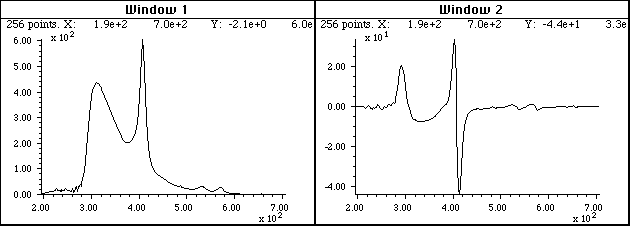A different application of Fourier deconvolution is to reveal the nature of an unknown data transformation function that has been applied to a data set by the measurement instrument itself. In this example, the figure in the top left is a uv-visible absorption spectrum recorded from a commercial photodiode array spectrometer (X-axis: nanometers; Y-axis: milliabsorbance). The figure in the top right is the first derivative of this spectrum produced by an (unknown) algorithm in the software supplied with the spectrometer. The objective here is to understand the nature of the differentiation/smoothing algorithm that the instrument's software uses. The signal in the bottom left is the result of deconvoluting the derivative spectrum (top right) from the original spectrum (top left). This therefore must be the convolution function used by the differentiation algorithm in the spectrometer's software. Rotating and expanding it on the x-axis makes the function easier to see (bottom right). Expressed in terms of the smallest whole numbers, the convolution series is seen to be +2, +1, 0, -1, -2.  This simple example of "reverse engineering" would make it easier to compare results from other instruments or to duplicate these result on other equipment.When applying Fourier deconvolution to experimental data, for example to remove the effect of a known broadening or low-pass filter operator caused by the experimental system, there are four serious problems that limit the utility of the method:

(1) the convolution occurring in the physical domain might not be accurately modeled by a mathematical convolution;

(2) the width of the convolution - for example the time constant of a low-pass filter operator or the shape and width of a spectrometer slit function - must be known, or at least adjusted by the user to get the best results;

(3) a serious signal-to-noise degradation commonly occurs; any noise added to the signal by the system after the convolution by the broadening or low-pass filter operator will be greatly amplified when the Fourier transform of the signal is divided by the Fourier transform of the broadening operator, because the high frequency components of the broadening operator (the denominator in the division of the Fourier transforms) are typically very small, with some individual components often of the order of 10-12 or 10-15, resulting a huge amplification of those particular frequencies in the resulting deconvoluted signal, which is called "ringing". (See the Matlab/Octave code example at the bottom of this page). The problem can be reduced either by low-pass filtering (smoothing) or even more simply by adding a small positive non-zero constant to the denominator, which increases the excessively small high-frequency members in the denominator without significantly increasing the much greater low-frequency members. Smoothing or filtering reduces the amplitude of the highest-frequency components, and denominator addition reduces the amplitude of the frequencies that are the most highly amplified by deconvolution. Both methods can have a similar effect, but they work in different ways and can sometimes be more effective when used together rather than separately.

You can see the amplification of high frequency noise happening in the example in the first example above. On the other hand, this effect is not observed in the second example, because in that case the noise was present in the original signal, before the convolution performed by the spectrometer's derivative algorithm. The high frequency components of the denominator in the division of the Fourier transforms are typically much larger than in the previous example, avoiding the noise amplification and divide-by-zero errors, and the only post-convolution noise comes from numerical round-off errors in the math computations performed by the derivative and smoothing operation, which is always much smaller than the noise in the original experimental signal.

In many cases, the width of the physical convolution is not known exactly, so the deconvolution must be adjusted empirically to yield the best results. Similarly, the width of the final smooth operation must also be adjusted for best results. The result will seldom be perfect, especially if the original signal is noisy, but it is often a better approximation to the real underlying signal than the recorded data without deconvolution.

As a method for peak sharpening, deconvolution can be compared to the derivative peak sharpening method described earlier or to the power method, in which the raw signal is simply raised to some positive power n.SPECTRUM, the freeware signal-processing application for Mac OS8 and earlier, includes a Fourier deconvolution function.Matlab and Octave have a built-in function for Fourier deconvolution: deconv. An example of its application is shown below: the vector yc (line 6) represents a noisy rectangular pulse (y) convoluted with a transfer function c before being measured. In line 7, c is deconvoluted from yc, in an attempt to recover the original y. This requires that the transfer function c be known. The rectangular signal pulse is recovered in the lower right (ydc), complete with the noise that was present in the original signal.  The Fourier deconvolution reverses not only the signal-distorting effect of the convolution by the exponential function, but also its low-pass noise-filtering effect. As explained above, there is significant amplification of any noise that is added after the convolution by the transfer function (line 5). This script demonstrates that there is a big difference between noise added before the convolution (line 3), which is recovered unmodified by the Fourier deconvolution along with the signal, and noise added after the convolution (line 6), which is amplified compared to that in the original signal. Execution time: 0.03 seconds in Matlab; 0.3 seconds in Octave.  Download this script.

x=0:.01:20;y=zeros(size(x));
y(900:1100)=1;                % Create a rectangular function y,
% 200 points wide

y=y+.01.*randn(size(y));      % Noise added before the convolution
c=exp(-(1:length(y))./30);    % exponential trailing convolution
% function, c

yc=conv(y,c,'full')./sum(c);  % Create exponential trailing rectangular
% function, yc

% yc=yc+.01.*randn(size(yc)); % Noise added after the convolution
ydc=deconv(yc,c).*sum(c);     % Attempt to recover y by deconvoluting c from yc
% The sum(c2) is included simply to scale the amplitude of the result to match the original y.
% Plot all the steps
subplot(2,2,1); plot(x,y); title('original y'); subplot(2,2,2); plot(x,c);title('c'); subplot(2,2,3); plot(x,yc(1:2001)); title('yc'); subplot(2,2,4); plot(x,ydc);title('recovered y')

Alternatively, you could perform the Fourier deconvolution yourself without using the built-in Matlab/Octave "deconv" function by dividing the Fourier transforms of yc and c using the built-in Matlab/Octave "fft.m" function and inverse transform the result with the built-in Matlab/Octave "ifft.m" function. Note that c must be zero-filled to match the size of yc. The results are essentially the same (except for the numerical floating point precision of the computer, which is usually negligible), and it's actually ten times faster than using the deconv function:

ydc=ifft(fft(yc)./fft([c zeros(1,2000)])).*sum(c);

Click here for a simple explicit example of Fourier convolution and deconvolution, for a small 9-element vector, with the vectors printed out at each stage.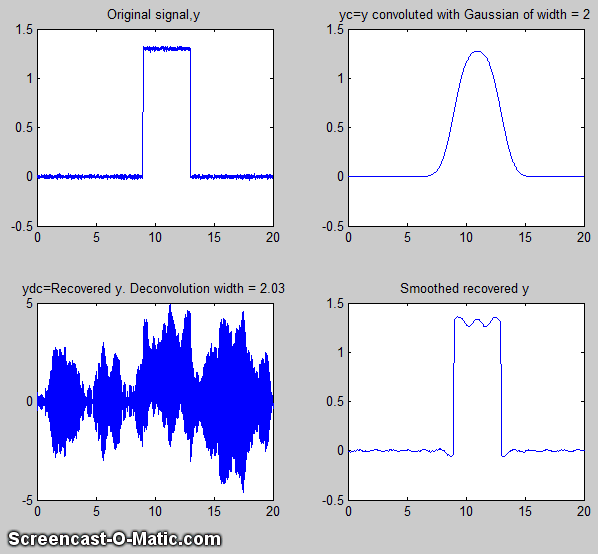The script DeconvDemo3.m is similar to the above, except that it demonstrates Gaussian Fourier convolution and deconvolution of the same rectangular pulse, utilizing the fft/ifft formulation just described. The animated screen graphic is shown on the left, demonstrating the effect of changing the deconvolution width. The raw deconvoluted signal in this example (bottom left quadrant) is extremely noisy, but that noise is mostly "blue" (high frequency) noise that is easily reduced by a little smoothing. As you can see in both of the animated examples here, deconvolution works best when the deconvolution width exactly matches the width of the convolution that the observed signal has been subject to; the further off you are, the worse will be the wiggles and other signal artifacts. In practice, you have to try several different deconvolution widths to find the one that results in the smallest wiggles, which of course becomes harder to see if the signal is very noisy.

DeconvDemo4.m (on the right) shows a Gaussian deconvoluted from a Gaussian function and an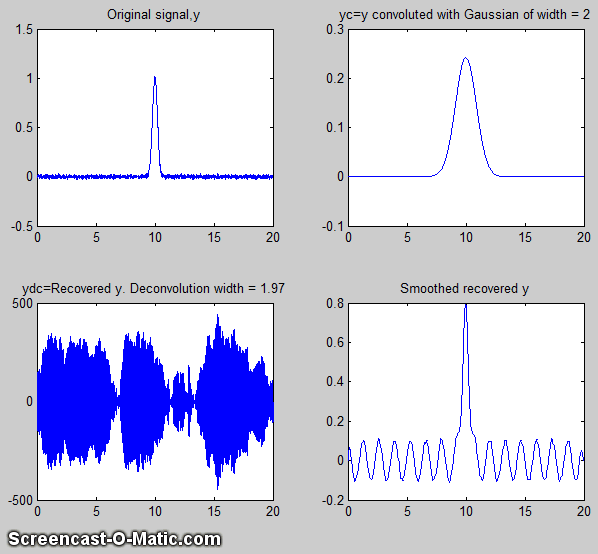attempt to recover the original peak width. Typically this would be applied to a signal containing multiple overlapping peaks, in an attempt to sharpen the peaks to improve the resolution. Note that in this example the deconvolution width must be within 1% of the deconvolution width. In general, the wider the physical convolution width relative to the signal, the more accurately the deconvolution width must be matched the physical convolution width.

DeconvDemo5.m(shown on the left) shows an example with two closely-spaced underlying peaks of equal width that are completely unresolved in the observed signal, but are recovered with their 2:1 height ratio intact in the deconvoluted and smoothed result. DeconvDemo6.m is that same except that the underlying peaks are Lorentzian. This image shows the widths of the peaks (as full width at half maximum) in the captions, showing that the width of the deconvoluted peaks (lower right quadrant) are actually slightly larger than in the (unobserved) underlying peaks (upper left quadrant) either because of imperfect deconvolution or because of the broadening effects of the smoothing needed to reduce the high frequency noise. (Note that all these scripts require functions than can be downloaded from http://tinyurl.com/cey8rwh).

In the example, shown below on the right, the underlying signal (uyy) is a Gaussian, but in the observed signal (yy) the peak is broadened exponentially resulting in a shifted, shorter, and wider peak, and then a little constant white noise is added after the broadening convolution (cc). Assuming that the exponential broadening time constant ('tc') is known, or can be guessed or measured, the Fourier deconvolution of cc from yy successfully removes the broadening (yydc), and restores the original height, position, and width of the underlying Gaussian, but at the expense of considerable noise increase.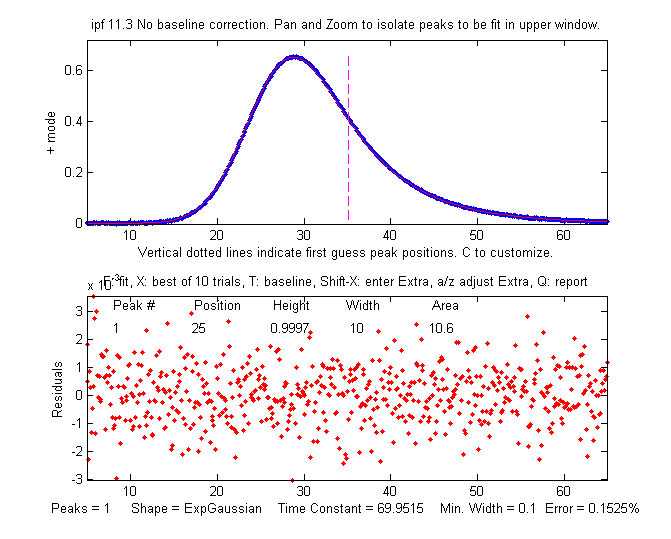However, the noise remaining in the deconvoluted signal is "blue" (high-frequency weighted) and so is easily reduced by smoothing and has less effect on least-square fits than does white noise. (For a greater challenge, try more noise in line 6 or a bad guess of time constant ('tc') in line 7). To plot the recovered signal overlaid with underlying signal: plot(xx,uyy,xx,yydc). To plot the observed signal overlaid with with underlying signal:

plot(xx,uyy,xx,yy).

To curve fit the recovered signal to a Gaussian to determine peak parameters: [FitResults,FitError]=peakfit([xx;yydc],26,42,1,1,0,10), which yields excellent values for the original peak positions, heights, and widths. You can demonstrate to yourself that with ten times the previous noise level (Noise=.01 in line 6), the values of peak parameters determined by curve fitting are still quite good, and even with 100x more noise (Noise=.1 in line 6) the peak parameters are more accurate than you might expect for that amount of noise (because that noise is blue). Remember, there is no need to smooth the results of the Fourier deconvolution before curve fitting, as seen previously.

% Deconvolution demo 2
xx=5:.1:65;
% Underlying signal with a single peak (Gaussian) of unknown
% height, position, and width.
uyy=gaussian(xx,25,10);

% Compute observed signal yy, using the expgaussian function with time
Noise=.001;  % <<<< Change the noise here
tc=70; % <<<< Change the exponential time constant here
yy=expgaussian(xx,25,10,-tc)'+Noise.*randn(size(xx));

% Guess, or use prior knowledge, or curve fit one peak, to
% determine time constant (tc), then compute transfer function cc
cc=exp(-(1:length(yy))./tc);

% Use "deconv" to recover original signal uyy by deconvoluting cc
% from yy. It's necessary to zero-pad the observed signal as shown here.
yydc=deconv([yy zeros(1,length(yy)-1)],cc).*sum(cc);

% Plot the signals and results in 4 quadrants
subplot(2,2,1);
plot(xx,uyy);title('Underlying 4 Gaussian signal, uyy');
subplot(2,2,2);
plot(xx,cc);title('Exponential transfer function, cc')
subplot(2,2,3);
plot(xx,yy);title('observed broadened and noisy signal, yy');
subplot(2,2,4);
plot(xx,yydc);title('After deconvoluting transfer function, yydc')

An alternative to the above deconvolution approach is to use iterative curve fitting (which is covered in a further section) to fit the observed signal directly with an exponentially broadened Gaussian (shape number 5):

>> [FitResults,FitError]=peakfit([xx;yy], 26, 50, 1, 5, 70, 10)

Both methods give good values of the peak parameters, but the Fourier deconvolution method is faster, because fitting the deconvoluted signal with a simple Gaussian model is faster than iteratively curve fitting the observed signal with the more complicated exponentially broadened Gaussian model. If the exponential factor "tc" is not known, it can be determined by iterative curve fitting, manually adjusting the exponential factor ('extra') interactively to get the best fit:

>> ipf([xx;yy]);

which in this particular case gives a best fit when the exponential factor "tc" is adjusted to about 69.9 (very close the correct value of 70 in this simulation).

Alternatively, you can use peakfit.m with the unconstrained variable exponentially broadened Gaussian (shape 31), which will automatically find the best value of "tc", but in that case the best results will be obtained if you give it a rough starting guess ("start") at least within a factor of two or so of the correct values:

>>[FitResults,FitError]=peakfit([xx;yy],0,0,1,31,70,10, [20 10 50])

FitResults =  Peak#   Position     Height       Width        Area           tc
1       25.006      0.99828       10.013       10.599        69.83
GoodnessOfFit =
0.15575      0.99998

The value of the exponential factor determined by this method is 69.8, again close to 70. However, if the signal is very noisy, there will be quite a bit of uncertainty in the value of the exponential factor so determined - for example, the value will vary a bit if slightly different regions of the signal are selected for measurement (e.g. by panning or zooming in ipf.m or by changing the center and window arguments in peakfit.m). See Appendix H: Fourier deconvolution vs curve fitting for another example with four overlapping Gaussians.

Self deconvolution. All the above example were aimed at removing known or suspected broadening effect that had caused the peaks to be broader that than the might otherwise have been. However, it is also possible to use the deconvolution technique to sharpen peaks that that have no known broadening function. This is an example of "self deconvolution", so-called because the shape of the deconvolution function is the same as the shape of the peaks in the signal.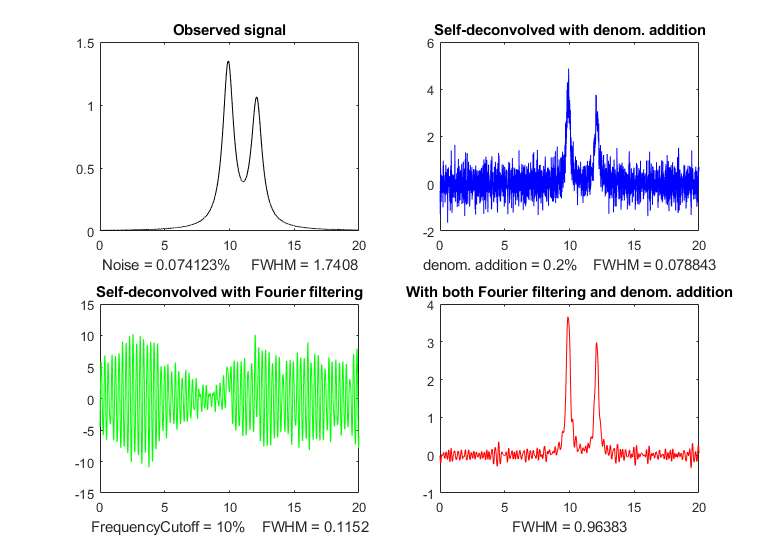For example, the figure on the right (Matlab script) shows the application of self deconvolution of two overlapping Lorentzian peaks with a narrower Lorentzian function, using a Fourier filter (next section) to reduce noise and ringing. Alternatively, and in addition to filtering, you can use the trick of adding a small constant to the denominator in the deconvolution division. The figure shows the observed signal (blue), the self deconvoluted peak with denominator addition (lower left), and self deconvoluted peak with Fourier filtering (lower right). Because this is self deconvolution, the shape of the peaks remains Lorentzian, but the widths are substantially narrowed, as the expense of degraded signal-to-noise ratio.

The Matlab script also displays the Fourier spectra of those four signals in their corresponding colors, in figure window 2, shown on the left. Observing these spectra can be a useful guide to adjusting the deconvolution width and the noise/ringing reduction settings. Looking at the spectrum of the original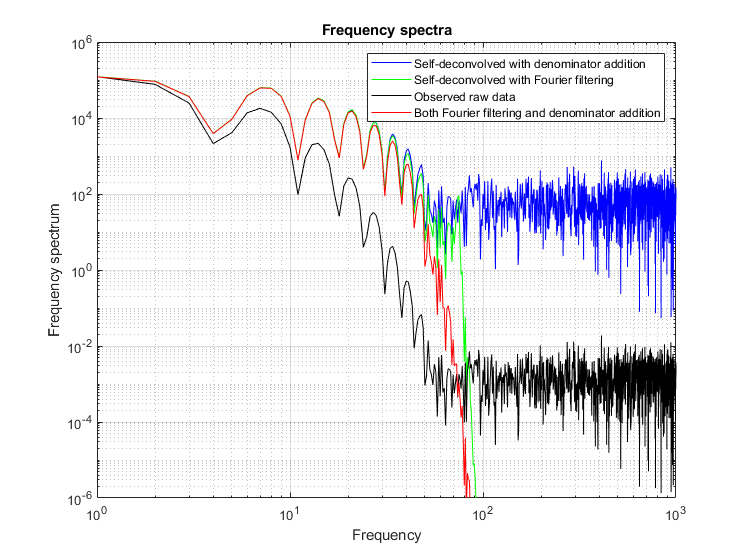raw data (black) you can see two distinct frequency regions:

(a) below a frequency of 102 there are a series of smooth descending humps, which are components of the Fourier transform of the signal peaks, and

(b) above 102 there is a flat jagged region - that's the high frequency end of the spectrum of the white noise in the signal, which is the same at all frequencies.

In order to sharpen the peaks, we need to raise the amplitude of the high-frequency components of the signal up to 102 and decrease the amplitude of the noisy region above 102.  The deconvolution process by itself increases all the high-frequency components of the signal, as you can see in the figure above left.

In all of the above simulations, the deconvolution method works as well as it does because the signal-to-noise ratio of the "observed signal" is quite good; the noise is not even visible on the scale presented here. In the absence of any knowledge of the width of the deconvolution function, finding the right deconvolution width depends upon experimentally minimizing the wiggles that are seen when the deconvolution width is incorrect, and a poor signal-to-noise ratio will make this much more difficult. Of course smoothing can reduce noise, especially high-frequency (blue) noise, but smoothing also slightly increases the width of peaks, which works counter to the point of deconvolution, so it must not be over used. As a rough but practical rule of thumb, if there is any easily visible noise in the observed signal, it is likely that the results of self-deconvolution, of the type shown in  DeconvDemo5.m, will be quite noisy.

Segmented deconvolution. If either the peak widths or the tailing varies substantially across the signal, you can use a segmented deconvolution, which allows the deconvolution vector to adapt to the local conditions in different signal regions. SegExpDeconv(x,y,tc) divides x,y into a number of equal-length segments defined by the length of the vector 'tc', then each segment is deconvoluted with an exponential decay of the form exp(-x./t) where t is corresponding element of the vector tc. Any number and sequence of t values can be used. SegExpDeconvPlot.m is the same except that it plots the original and deconvoluted signals and shows the divisions between the segments by vertical magenta lines to make it easier to adjust the number and values of the segments. SegGaussDeconv.m and SegGaussDeconvPlot.m are the same except that they perform a symmetrical (zero-centered) Gaussian deconvolution. SegDoubleExpDeconv.m and SegDoubleExpDeconvPlot.m perform a symmetrical (zero-centered)exponential deconvolution. If the peak widths increase regularly across the signal, you can calculate a reasonable initial value for the vector 'tc' by giving only the number of segments (NumSegments) , the first value, "startt", and the last value, "endt":

tstep=(endt-startt)/NumSegments;

tc=startt:tstep:endt;

Combination function. The Matlab/Octave function P=convdeconv(x,y,vmode,smode,vwidth,DAdd) performs Gaussian, Lorentzian, or exponential convolution and deconvolution of the signal in x,y. Set vmode=1 for convolution, 2 for deconvolution, smode=1 for Gaussian, 2 for Lorentzian, 3 for exponential; vwidth is the width of the convolution or deconvolution function, and DAdd is the constant denominator addition used to control ringing and noise resulting from deconvolution. For examples of the operation of this function, see ThreeGaussianSignalTrial.m and ThreeLorentzianSignalTrial.m. You can clearly see the substantial increase in peak height and decrease in peak width resulting from deconvolution. Although the peak heights are increased (by deconvolution) and the peak areas are slightly reduced (by the denominator addition), that will not be a problem in quantitative analysis by calibration curves as long as the same deconvolution setting are used for all samples and standards. The random noise is also increased; nevertheless, the peak heights, positions, and widths can still be measured precisely by least squares methods. The noise can be reduced by applying a little subsequent smoothing. That leaves the question of which values of vwidth and DAdd to use. Start with a value of vwidth somewhat smaller than the estimated width of the peaks in the signal, with DAdd set to zero or some small number like 0.001%, then try larger values of vwidth to increase the peak sharpening. If noise and ringing starts to obscure the signal, try larger values of DAdd. Excessively large values of either can distort the peaks.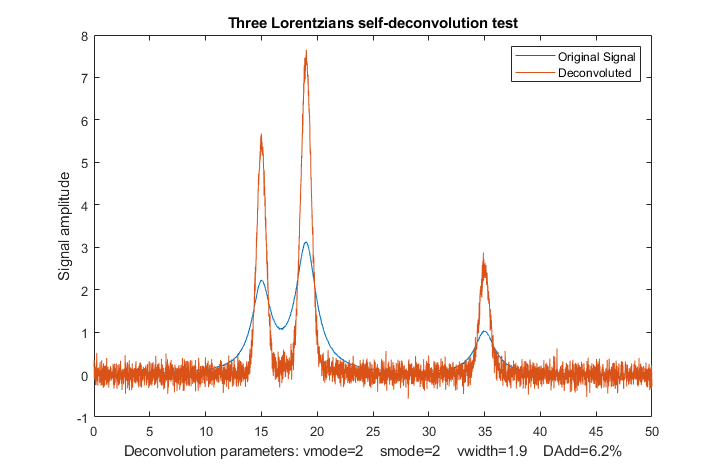-----------------------------------------
Parameters of the three Lorentzian peaks
Peak      Height     FWHM      Area
1.0000    2.0000    2.0000    6.0929
2.0000    3.0000    2.2000   10.0594
3.0000    1.0000    2.2000    3.3407

Parameters of the three deconvoluted peaks
Peak      Height     FWHM      Area
1.0000    5.6963    0.9624    5.9684
2.0000    7.6741    1.0711    9.3350
3.0000    2.7056    0.8623    3.0578
-----------------------------------------

In iSignal version 8.3, for Matlab only, the downloadable interactive multipurpose signal processing Matlab functionyou can press Shift-V to display the menu of Fourier convolution and deconvolution operations that allow you to convolute or to deconvolute a Gaussian, Lorentzian or exponential function. It will ask you for the initial width or time constant of the deconvolution function (in X units), then you can use the 3 and 4 keys to decrease or increase the width by 10% (or Shift-3 and Shift-4 to adjust by 1%). This version of iSignal includes an additional way to reduce ringing and noise in the deconvoluted signal, by adding a constant to the denominator (reference 86) and adjusting it with the 5 and 6 keys to decrease or increase the constant by 10% (or Shift-5 and Shift-6 to adjust by 1%). Here's an application to a real experimental signal: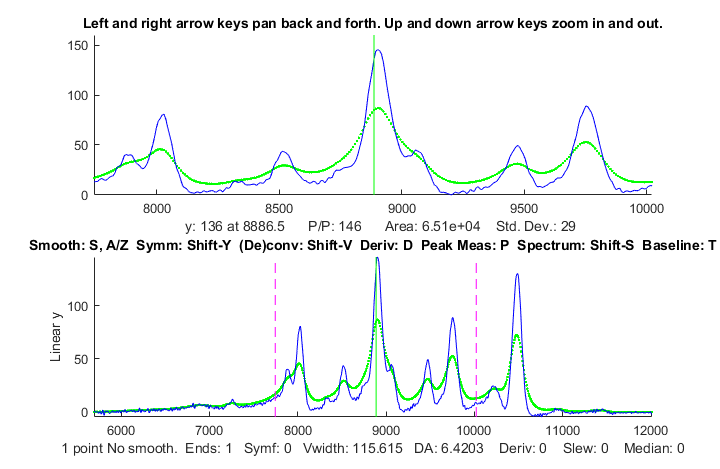In this example, the original signal is shown as the dotted green line and the results of deconvoluting it with a Lorentzian deconvolution function is shown as the blue line. The deconvolution width was adjusted as large as possible without causing significant negative dips between the peaks, which for many types of experimental data, would be non-physical. (Recall that the mathematics of the deconvolution operation is structured so that the area under the peaks remains unchanged, even though the widths are reduced and the heights are increased). Several of the peaks shown in the zoomed-in close-up in the upper panel have shoulders that are resolved into distinct peaks, allowing their peak positions to be measured more accurately. Fortunately, the amplitude of those revealed peaks is greater than the small amount of noise remaining in the signal (thanks to the good signal-to-noise ratio of the original signal).

An older version of this page is also available in French, at http://www.besteonderdelen.nl/blog/?p=41, courtesy of Natalie Harmann.Revised June, 2020. This page is part of "A Pragmatic Introduction to Signal Processing", created and maintained by Prof. Tom O'Haver , Department of Chemistry and Biochemistry, The University of Maryland at College Park. Comments, suggestions and questions should be directed to Prof. O'Haver at toh@umd.edu.
Unique visits since May 17, 2008: